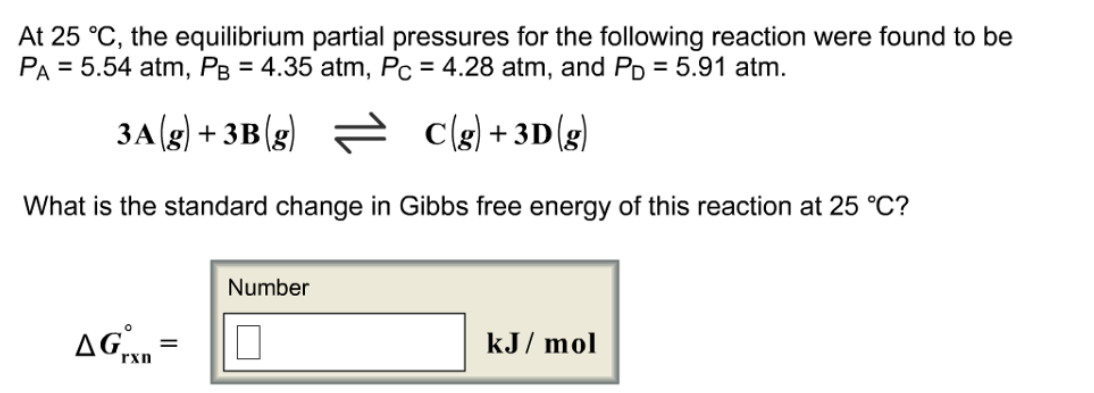# Problem: At 25 °C, the equilibrium partial pressures for the following reaction were found to be PA = 5.54 atm, PB = 4.35 atm, Pc = 4.28 atm, and PD = 5.91 atm.3A(g) + 3B(g) → C(g) + 3D (g)What is the standard change in Gibbs free energy of this reaction at 25 °C?

###### FREE Expert Solution
81% (111 ratings)###### Problem Details

At 25 °C, the equilibrium partial pressures for the following reaction were found to be PA = 5.54 atm, PB = 4.35 atm, Pc = 4.28 atm, and PD = 5.91 atm.

3A(g) + 3B(g) → C(g) + 3D (g)

What is the standard change in Gibbs free energy of this reaction at 25 °C?